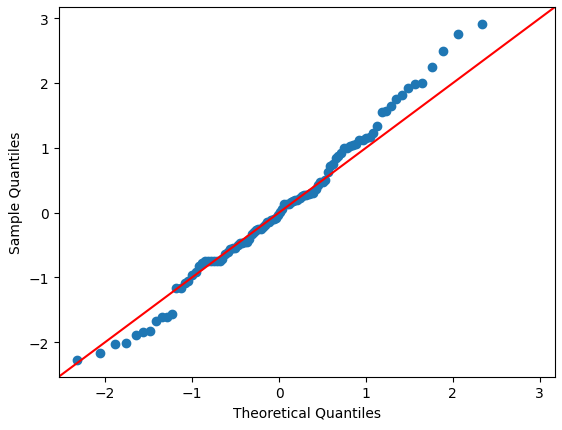# How to show a QQ-plot?

I want to show a QQ-plot in a Holoviz panel. Normally I would create a QQ-plot as shown below:

``````import statsmodels.api as sm
sm.qqplot(df.cost, fit=True, line ='45', dist=stats.invgauss);
``````

However, no matter what I do I can not get the resulting figure to show in a Holoviz panel. How do I get the QQ-plot to show?

1 Like

Could you provide a minimum, reproducible example? That would make the investigation much easier? Thanks.

Here is a small code example in which random data is produced and plotted:

``````import statsmodels.api as sm
import numpy as np

data = np.random.normal(0, 1, 100)
sm.qqplot(data, line ='45');
``````

This produces the output:Hi. Panel needs to receive a Matplotlib Figure object. C.f. Matplotlib — Panel.

Very often functions that plot via Matplotlib accept an `ax` argument where you can then provide the Matplotlib `Axes` object to plot on. You can create the `Axes` from the `Figure` object as shown below.

``````import statsmodels.api as sm
import numpy as np
from matplotlib.figure import Figure
from matplotlib import cm

def qqplot(data, line):
fig = Figure(figsize=(8, 6))
ax = fig.subplots()
sm.qqplot(data, line=line, ax=ax)

return fig

data = np.random.normal(0, 1, 100)

import panel as pn

pn.extension(sizing_mode="stretch_width", design="bootstrap")
line = pn.widgets.Select(name="Line", value="45", options=["45", "s", "r", "q", None])

plot = pn.bind(qqplot, line=line, data=data)

pn.Column(
line,
pn.pane.Matplotlib(plot, tight=True, format="svg"),
max_width=800,
).servable()

``````

1 Like

Thank you so much! I was wondering if it would be possible to bind the argument `dist` from `sm.qqplot(..., dist=...)` to a button?

1 Like

I don’t understand what you mean by button.

But with the help of `Param` and `Panel` you can make the `qqplot` interactive. Including the `dist` parameter.

``````import statsmodels.api as sm
import numpy as np
from matplotlib.figure import Figure

from param import Parameterized
import param
import scipy.stats as stats

class QQPlot(Parameterized):
"""Function to draw qqplot"""

data = param.Parameter(precedence=-1)
line = param.Selector(objects=["45", "s", "r", "q", None])
dist = param.Selector(objects={"None": None, "T-distribution": stats.t})
distargs = param.Tuple(default=(1,))

def fig(self):
_fig = Figure(figsize=(8, 6))
ax = _fig.subplots()

if self.dist:
sm.qqplot(
data=self.data,
line=self.line,
dist=self.dist,
distargs=self.distargs,
ax=ax,
)
else:
sm.qqplot(data=self.data, line=self.line, ax=ax)
return _fig

@param.depends("dist", watch=True)
def _hide_distargs(self):
if self.dist:
self.param.distargs.precedence = None
else:
self.param.distargs.precedence = -1

data = np.random.normal(0, 1, 100)

plot = QQPlot(data=data, dist=stats.t, distargs=(4,))

import panel as pn

pn.extension(sizing_mode="stretch_width", design="bootstrap")

pn.Row(
pn.WidgetBox(plot, sizing_mode="fixed", width=300),
pn.panel(plot.fig, tight=True),
margin=25,
).servable()
``````

Do you have any idea why the toggles do not have an effect in the code below:

``````def qqplot(data, distribution):
fig = Figure(figsize=(8, 6))
ax = fig.subplots()
sm.qqplot(data,  fit=True, line ='45', dist=distribution, ax=ax)
return fig

toggles = pn.widgets.ToggleGroup(
name="Select distribution",
options=["Inverse Gaussian", "Normal", "Binomial", "Gamma"],
value="Normal",
)

distributions = pd.Series([stats.invgauss, stats.norm, stats.binom, stats.gamma],
index=["Inverse Gaussian", "Normal", "Binomial", "Gamma"])
fig_QQ = pn.bind(qqplot, data=df.income, distribution=distributions[toggles.value])
``````

The plot does not react to changes in the toggles. I get the following warning as well:

``````WARNING:param.ParamFunction01034: The function 'qqplot' does not have any dependencies and will      never update. Are you sure you did not intend to depend on or bind a parameter or widget to this function? If not simply call the function before passing it to Panel. Otherwise, when passing a parameter as an argument, ensure you pass at least one parameter and reference the actual parameter object not the current value, i.e. use object.param.parameter not object.parameter
``````

Yes. Its the line `distributions=distributions[toggles.value]` that does not make sense.

Try reorganizing to

``````def qqplot(data, distribution_name):
distribution = distributions[distribution_name]
fig = Figure(figsize=(8, 6))
ax = fig.subplots()
sm.qqplot(data,  fit=True, line ='45', dist=distribution, ax=ax)
return fig

toggles = pn.widgets.ToggleGroup(
name="Select distribution",
options=["Inverse Gaussian", "Normal", "Binomial", "Gamma"],